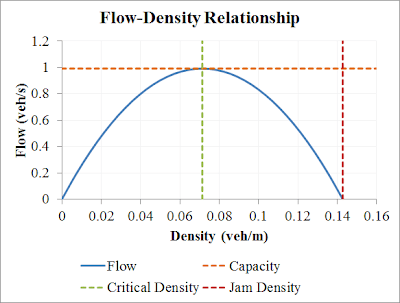## Saturday 8 December 2012

### Analysis of Speed Density Flow Relationship

These notes have been inspired by a recent paper by Rahman, Ben-Edigbe and Hassan*. In it they analyse the speed, density and flow relationship.

The equations given in this previous traffic modeling post include an algebraic relationship between speed, density and flow: $$q=\rho v$$ and $$v = v_f(1-\rho/\rho_j),$$ where
$$q$$ = flow rate (veh/s);
$$\rho$$ = vehicle density (veh/m);
$$v$$ = vehicle speed (m/s);
$$v_f$$ = free flow speed (m/s), nominally 27.78 m/s;
$$\rho_j$$ = jam density (veh/m), nominally 1/7 veh/m.

Given the above equations, it is possible to eliminate $$v$$. That is, flow can be expressed in terms of density by using the speed equation:
$$q=v_f\rho ( 1 - \rho/\rho_j ).$$ This is a quadratic, and it has a maximum. Because it's a quadratic, this means that the inverse relationship is not a function, as it's multi-valued (one flow rate may be associated with two densities). Here is a graph of the flow-density relationship with the nominal values:Relationship between flow and density, showing key values.

The maximum point on the flow curve happens to be the capacity of the roadway. We find the maximum point by differentiating the above and solving for slope equals zero. The critical density, $$\rho_c$$ (the density at which the flow rate equals capacity), is found to be $$\rho_j/2$$, and the capacity, $$q_c$$, is given by:
$$q_c=v_f\rho_j/4.$$ With the values mentioned above ($$v_f$$ = 27.78 and $$\rho_j$$ = 1/7), the capacity is 0.99 veh/s, which while high, is in the right order of magnitude for freeways (which I think is around 0.5 to 0.7 veh/s).

The next post on this topic will be model discretisation.

* The paper is Rahman, R., Den-Edigbe, J. E., and Hassan A. (2012), Extent of Traffic Shockwave Velocity Propagations Induced by U-Turn Facility on Roadway Segments. 25th ARRB Conference. Mentioned here.